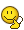# Finding velocity and position of ##a(t)=−\omega^2(C_1\cos\theta+C_2\sin\theta)##

• fer Mnaj
fer Mnaj
Homework Statement
Given this expression : ##a(t)=−\omega^2(C_1\cos \theta+C_2\sin\theta)##

And with two initial conditions ##x(0)=x_0## and ##v(0)=v_0##

How to get velocity and position?

Furthermore, Can we get the values of ##C_1## and ##C_2##, what are these?
Relevant Equations
centripetal aceleration is the most likely ##a(t)=−\omega^2(\cos\theta+\sin\theta)##
this expression : ##a(t) = −ω^2 (C_1 \cos θ + C_2 \sin θ)##
I´ve never seen it before, where is it from?

It kinda looks like centripetal acceleration, but what exactly are ##C_1## and ##C_2##?

Can we calculate its velocity and position?

If I´ve got two initial conditions ##x(0)=x_0## and ##v(0)=v_0##

I thought we could get velocity and position
with:

##\omega=\theta/t## IS constant

##dv/dt= −ω^2 (C_1 \cos θ + C_2 \sin θ)##

##\int dv=\int−ω^2 (C_1 \cos \omega t + C_2 \sin \omega t)dt##

##-\int1/w^2 dv=C_1\int \cos (\omega t) dt+ C_2\int\sin (\omega t) dt## is it correct?

Is it solvable?

Last edited:

Gold Member
It seems all right. Do integration.

fer Mnaj
What should it go in each side of the equation? velocity and position??

Gold Member
Velocity. Solve it first. Then in order to get position do time integration again.

Does the original expression have any vectors, that you might have omitted when writing it up? In it's current form its not clear what ##a(t)## refers to.

Staff Emeritus
Homework Helper
It looks like a one-dimensional simple harmonic oscillator problem.

•etotheipi
It looks like a one-dimensional simple harmonic oscillator problem.

Yes, you’re right. When I looked at it this morning I initially thought ##\theta## was a polar angle or something, for a particle moving in a weird force field, but yes it makes much more sense that it is actually the phase angle 🙂. Explains the ##-\omega^2## out the frontfer Mnaj
I also noticed it was the simple harmonic oscillator, but usually its solved from position to acceleration. Its taking me too much time to figure out in the opposite way

@fer Mnaj if you think about the general form for the equation of motion for a harmonic oscillator, you should be able to write down the function ##x(t)##.

But you can also just integrate the expression for ##a(t)## twice (do you know how to integrate trig functions?)

fer Mnaj
So I left it in: ##-\int1/w^2 dv=C_1\int \cos (\omega t) dt+ C_2\int\sin (\omega t) dt##
now let ##\omega t=u## so ##du/dt=\omega## and ##dt=du/\omega##
##-1/w^2\int dv=C_1\int \cos (u) du/\omega## + ##C_2\int\sin (u) du/\omega##
##-1/w^2\int dv=C_1/\omega\int \cos (u) du/## + ##C_2/\omega\int\sin (u) du##
##-1/w^2\int dv=C_1\omega^-1 sin(u)## - ##C_2\omega^-1 cos(u)##
##-1/w^2\int dv=C_1\omega^-1 sin(\omega t)## - ##C_2\omega^-1 cos(\omega t)##
Am I going right? what's next?

fer Mnaj
I missed the integration constants... how to aply them with the initial conditions i gave aa the begining?

Gold Member
$$-\frac{1}{\omega^2}[v]^v_{v_0}=\frac{C_1}{\omega}[sin\omega t]^t_0 - \frac{C_2}{\omega}[cos\omega t]^t_0$$
$$-\frac{1}{\omega^2}v_0=- \frac{C_2}{\omega}$$
So you can find the value of ##C_2##. Then further
$$-\frac{1}{\omega^2}\frac{dx}{dt}=\frac{C_1}{\omega}sin\omega t- \frac{C_2}{\omega}cos\omega t$$

Mentor
$$-\frac{1}{\omega^2}[v]^v_{v_0}=\frac{C_1}{\omega}[sin\omega t]^t_0 - \frac{C_2}{\omega}[cos\omega t]^t_0$$
$$-\frac{1}{\omega^2}v_0=- \frac{C_2}{\omega}$$
So you can find the value of ##C_2##. Then further
$$-\frac{1}{\omega^2}\frac{dx}{dt}=\frac{C_1}{\omega}sin\omega t- \frac{C_2}{\omega}cos\omega t$$
This is incorrect.

I'm actually shocked that no one here has yet been able to correctly integrate this simple first order ODE subject to an initial condition. The correct solution for the velocity is $$v=\omega(C_2 \cos{\omega t}-C_1\sin{\omega t})+C$$ where C is the constant of integration. Substituting the initial condition into this gives: $$v_0=\omega C_2+C$$So the constant of integration is:
$$C=v_0-\omega C_2$$. And thus the velocity is:
$$v=(v_0-\omega C_2)+\omega(C_2 \cos{\omega t}-C_1\sin{\omega t})$$

Last edited:
•anuttarasammyak